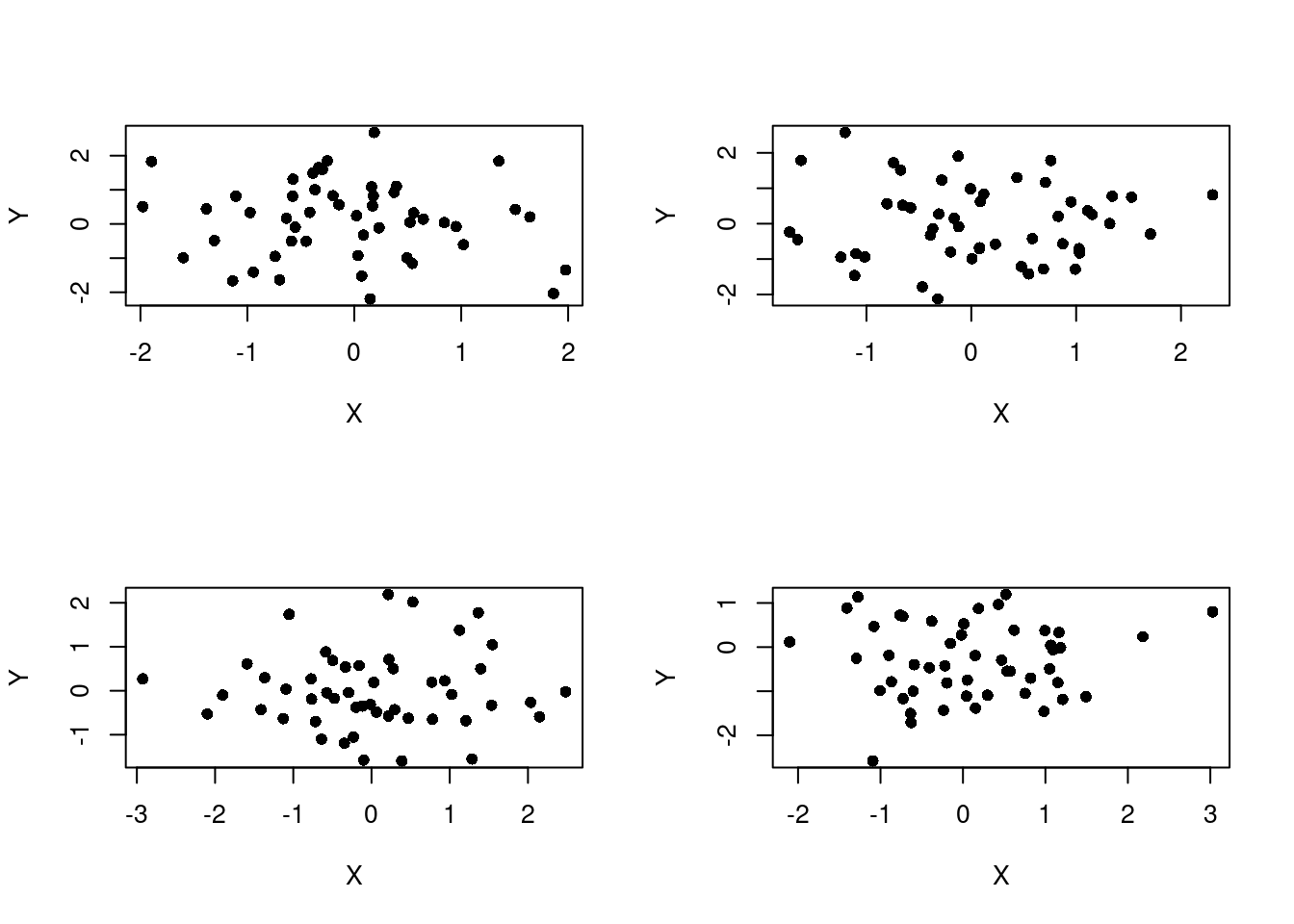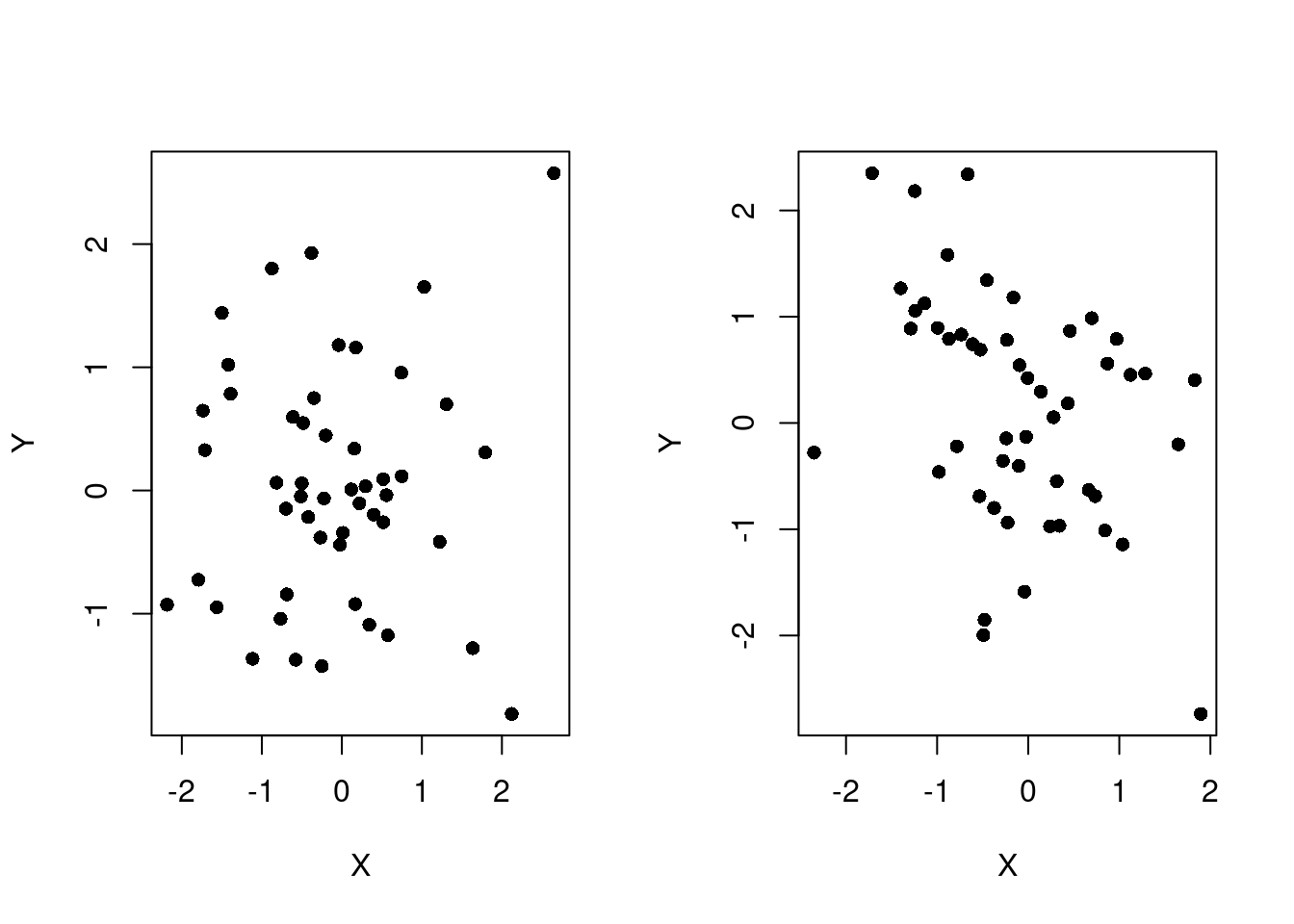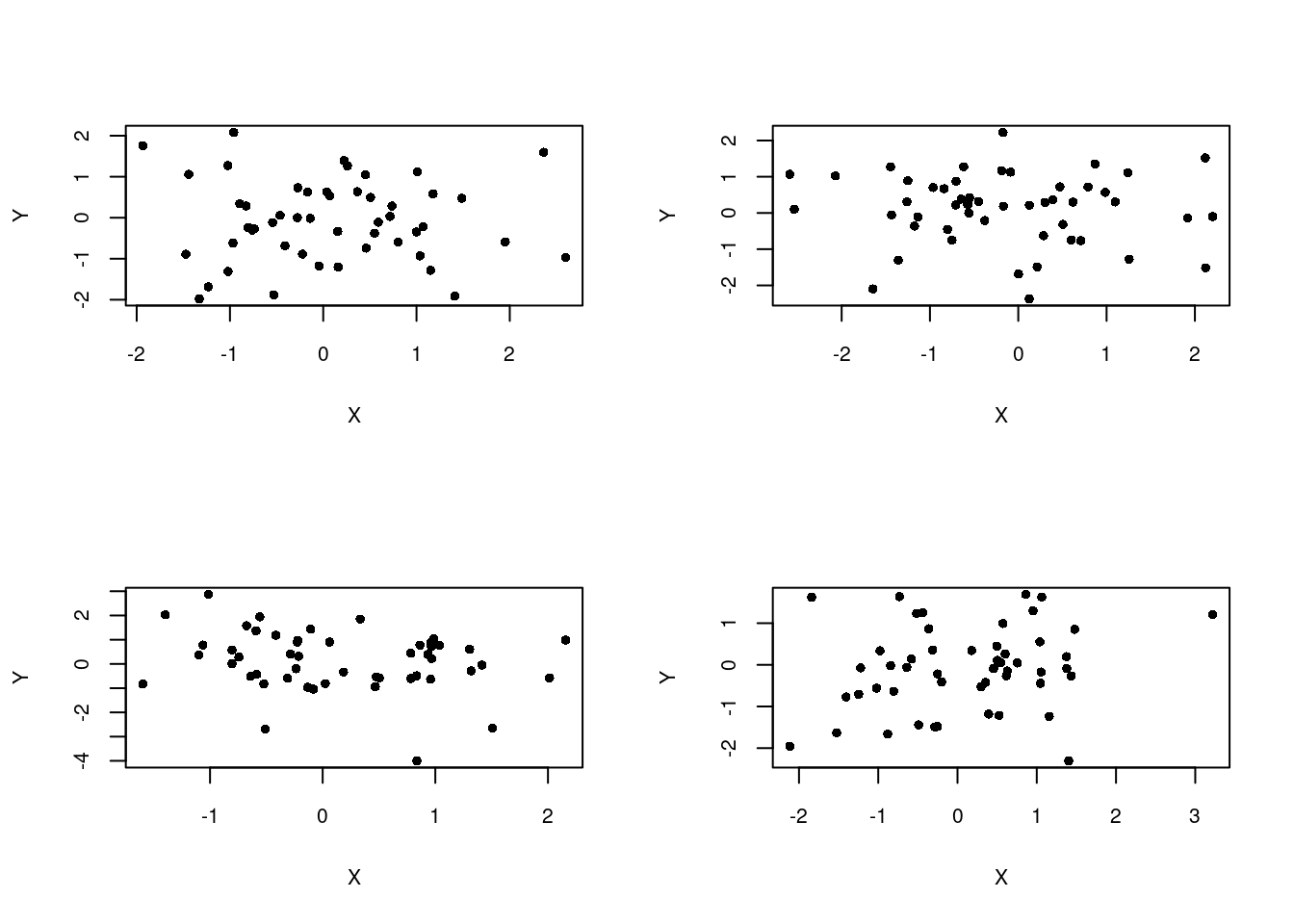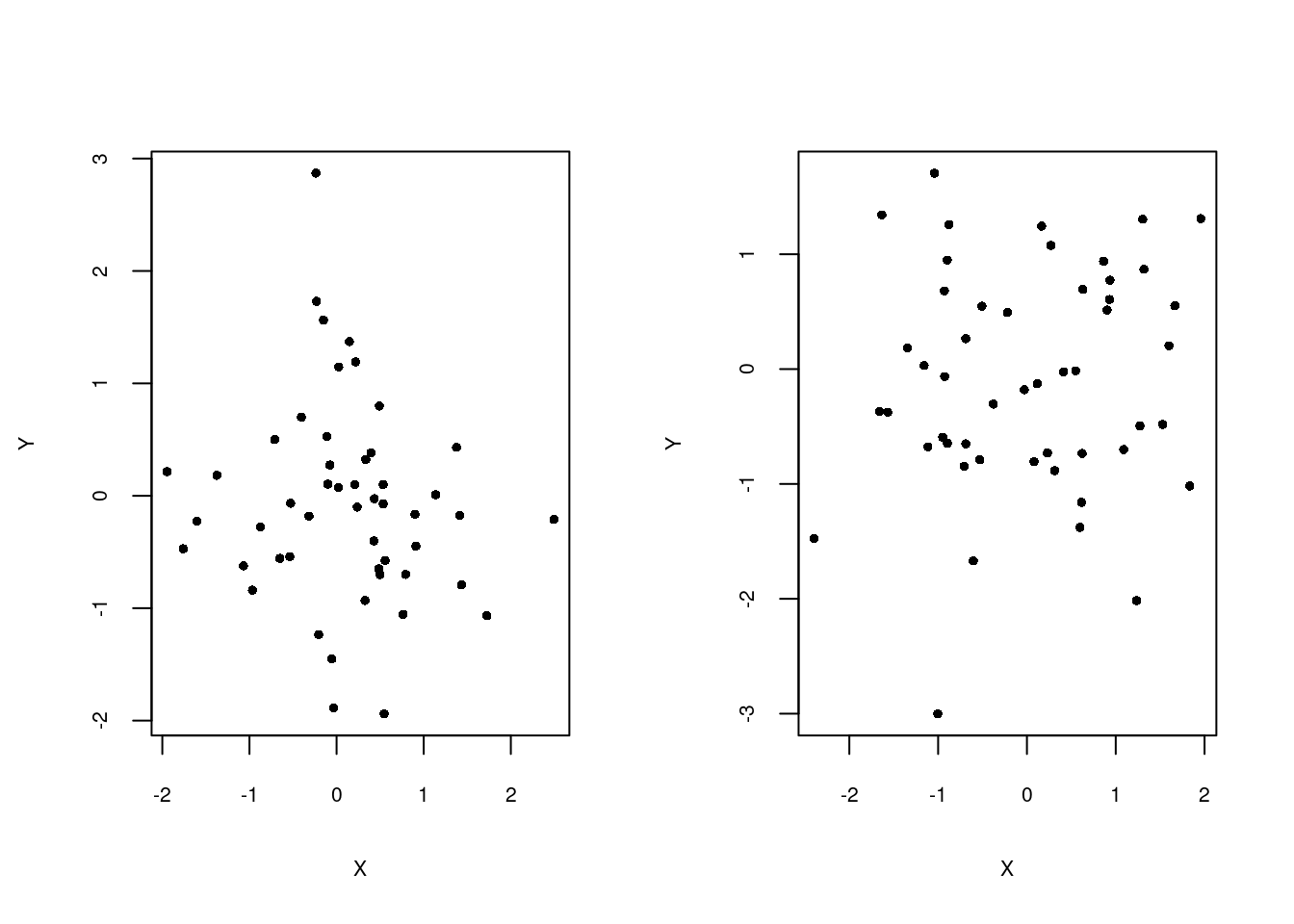# Text and symbol size in multi-panel figures in R

In R, there are a couple of packages that allow you to create multi-panel figures (ggplot2 and lattice), but, of course, you can also make multi-panel figures in the base package. Below, I provide a simple example for creating a multi-panel figure in the R base package with the focus on making the text and symbols the same size in all of your figures, which is a desirable trait for a set of figures that will appear in the same manuscript.

The `mfrow` argument of the `par` function determines the arrangement of the plots in the graphics device by specifying the number of rows and columns and the order that the graphics device is filled, i.e., by row (use `mfcol` to fill by columns). Below is example code and the figure that is produced from the code.

``````par(mfrow=c(3,2))
plot(rnorm(50),rnorm(50),pch=16,xlab="X",ylab="Y")
plot(rnorm(50),rnorm(50),pch=16,xlab="X",ylab="Y")
plot(rnorm(50),rnorm(50),pch=16,xlab="X",ylab="Y")
plot(rnorm(50),rnorm(50),pch=16,xlab="X",ylab="Y")
plot(rnorm(50),rnorm(50),pch=16,xlab="X",ylab="Y")
plot(rnorm(50),rnorm(50),pch=16,xlab="X",ylab="Y")``````Because the graphics device holds many plots, R automatically reduces the size of the text and symbols. From the R documentation (enter `?par` in the R console)…

In a layout with exactly two rows and columns the base value of `cex` is reduced by a factor of 0.83: if there are three or more of either rows or columns, the reduction factor is 0.66.

Thus, the size of the text and symbols in the figure above have been reduced by 66%. Below is an example with exactly two rows and columns to demonstrate the 83% reduction.

``````par(mfrow=c(2,2))
plot(rnorm(50),rnorm(50),pch=16,xlab="X",ylab="Y")
plot(rnorm(50),rnorm(50),pch=16,xlab="X",ylab="Y")
plot(rnorm(50),rnorm(50),pch=16,xlab="X",ylab="Y")
plot(rnorm(50),rnorm(50),pch=16,xlab="X",ylab="Y")``````And, finally, an example without any reduction in the size of text and symbols.

``````par(mfrow=c(1,2))
plot(rnorm(50),rnorm(50),pch=16,xlab="X",ylab="Y")
plot(rnorm(50),rnorm(50),pch=16,xlab="X",ylab="Y")``````To keep the text and symbol size the same across all the figures, we need to adjust the `cex` argument to account for the default adjustments made by `mfrow`. As an example, we will reduce the text/symbol size to match the 3x2 panel figure. However, when you know the adjustment factor of `mfrow`, you can adjust the size to match a figure with any number of panels.

We know from the documentation that a 2x2 figure involves a reduction of 83% relative to the default. We need to reduce the size of text/symbols by another 79.5% to achieve the same reduction as in the 3x2 figure (`0.83 * 0.795=0.66`), which is applied to the numbers on the axis (`cex.axis`), axis labels (`cex.lab`), and symbols (`cex`).

``````par(mfrow=c(2,2),cex.axis=0.795,cex.lab=0.795)
plot(rnorm(50),rnorm(50),pch=16,xlab="X",ylab="Y",cex=0.795)
plot(rnorm(50),rnorm(50),pch=16,xlab="X",ylab="Y",cex=0.795)
plot(rnorm(50),rnorm(50),pch=16,xlab="X",ylab="Y",cex=0.795)
plot(rnorm(50),rnorm(50),pch=16,xlab="X",ylab="Y",cex=0.795)``````A figure that has only one row has not been reduced by `mfrow` and, thus, should be reduced by 66% to match the reduction in the 3x2 figure.

``````par(mfrow=c(1,2),cex.axis=0.66,cex.lab=0.66)
plot(rnorm(50),rnorm(50),pch=16,xlab="X",ylab="Y",cex=0.66)
plot(rnorm(50),rnorm(50),pch=16,xlab="X",ylab="Y",cex=0.66)``````The figures in this post involve the default spacing produced by `mfrow`. In another `post`, I show which graphical arguments to change to make more attractive multi-panel figures with `mfrow`. Other options for multi-panel figures in the R base package include `layout` and `split.screen`.